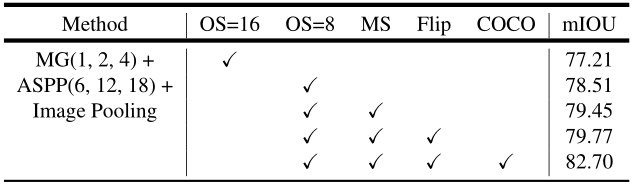# DeepLabV3深入解读¶

## 1、DeepLab系列简介¶

### 1.1.DeepLabV1¶

#### 1.1.1创新点：¶

• 将深度神经网络DCNN与全连接CRF结合起来，提高图像分割的分割精度。

• 提出空洞卷积的思想。

• 应用尝试了多尺度、多层次的信息融合。

#### 1.1.2. 动机：¶

DCNN应用在语义分割任务上存在两个缺陷：

• 重复堆叠的池化下采样操作导致分辨率大幅下降，位置信息丢失难以恢复。

• 从分类器获得以对象为中心的决策需要空间转换的不变性，忽略对细节处的把控，这从本质上限制了DCNN模型的空间准确性。

#### 1.1.3. 应对策略：¶

• 空洞卷积

• Fully-connected Conditional Random Field (CRF)

### 1.2.DeepLabV2¶

#### 1.2.1.创新点：¶

• 空洞卷积，作为密集预测任务的强大工具。空洞卷积能够明确地控制DCNN内计算特征响应的分辨率。它还允许我们有效地扩大滤波器的视野以并入较大的上下文，而不增加参数的数量或计算量。

• 提出了空洞空间卷积池化金字塔(atrous spatial pyramid pooling (ASPP))，以多尺度的信息得到更精确的分割结果。ASPP并行的采用多个采样率的空洞卷积层来探测，以多个比例捕捉对象以及图像上下文。

• 通过组合DCNN和概率图模型（CRF），改进分割边界结果。在DCNN中最大池化和下采样组合实现可平移不变性，但这对精度是有影响的。通过将最终的DCNN层响应与全连接的CRF结合来克服这个问题。

#### 1.2.2.动机¶

DCNNs中语义分割存在三个挑战：

• 连续下采样和池化操作，导致最后特征图分辨率低。

• 图像中存在多尺度的物体（相比V1而言提出的新的挑战

• 空间不变性导致细节信息丢失

#### 1.2.3. 应对策略：¶

• 移除部分池化操作，使用空洞卷积。

• 利用不同膨胀因子的空洞卷积融合多尺度信息—atrous spatial pyramid pooling(ASPP)（新的创新点

• 全连接CRF。

### 1.3.DeepLabV3¶

#### 1.3.1创新点：¶

• 增加了多尺度(multiple scales)分割物体的模块

• 设计了串行和并行的空洞卷积模块，采用多种不同的atrous rates（采样率）来获取多尺度的内容信息

#### 1.3.2. 动机：¶

DCNN中语义分割存在三个挑战：

• 连续下采用和重复池化，导致最后特征图分辨率低

• 图像中存在多尺度的物体

#### 1.3.3. 应对策略：¶

• 使用空洞卷积，防止分辨率过低情况

• 串联不同膨胀率的空洞卷积或者并行不同膨胀率的空洞卷积（v2的ASPP），来获取更多上下文信息

#### 1.3.4. 主要贡献：¶

• 重新讨论了空洞卷积的使用，这让我们在级联模块和空间金字塔池化的框架下，能够获取更大的感受野从而获取多尺度信息

• 改进了ASPP模块：由不同采样率的空洞卷积和BN层组成，我们尝试以级联或并行的方式布局模块

• 讨论了一个重要问题：使用大采样率的3×3的空洞卷积，因为图像边界的原因无法捕捉远距离信息，会退化为1×1的卷积, 作者提出将图像级特征融合到ASPP模块中

• 阐述了训练细节并分享了训练经验，论文提出的”DeepLabv3”改进了以前的工作，获得了很好的结果

### 1.4.DeepLabV3+¶

#### 1.4.1.创新点：¶

• 更深的Xception结构，不同的地方在于不修改entry flow network的结构，为了快速计算和有效的使用内存

• 所有的max pooling结构被stride=2的深度可分离卷积代替

• 每个3x3的depthwise convolution都跟BN和Relu

• 将改进后的Xception作为encodet主干网络，替换原本DeepLabv3的ResNet101

#### 1.4.2. 动机：¶

• 物体的多尺度问题（DeepLabV3解决）

• DCNN的多次下采样会造成特征图分辨率变小，导致预测精度降低，边界信息丢失（DeepLabV3+解决目标）

#### 1.4.3. 应对策略：¶

• 改进Xception，层数增加

• 将所有最大值池化层替换为带步长的深度可分离卷积层

## 2、DeepLabV3详解¶

### 2.1.提出问题¶

• 第一个挑战：连续池化操作或卷积中的stride导致的特征分辨率降低。这使得DCNN能够学习更抽象的特征表示。然而，这种不变性可能会阻碍密集预测任务，因为不变性也导致了详细空间信息的不确定。为了克服这个问题，提倡使用空洞卷积。

• 第二个挑战：多尺度物体的存在。几种方法已经被提出来处理这个问题，在本文中我们主要考虑了这些工作中的四种类型，如图1所示。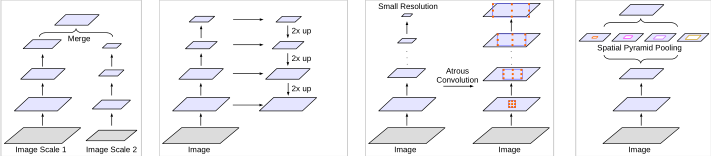• 第一种：Image Pyramid，将输入图片放缩成不同比例，分别应用在DCNN上，将预测结果融合得到最终输出

• 第二种：Encoder-Decoder，将Encoder阶段的多尺度特征运用到Decoder阶段上来恢复空间分辨率

• 第三种：在原始模型的顶端叠加额外的模块，以捕捉像素间长距离信息。例如Dense CRF，或者叠加一些其他的卷积层

• 第四种：Spatial Pyramid Pooling空间金字塔池化，使用不同采样率和多种视野的卷积核，以捕捉多尺度对象

DeepLabV3的提出是为了解决多尺度下的目标分割问题。如图2所示，不同目标在图中的尺寸大小不同，这也导致模型考虑不同尺寸的分割精度。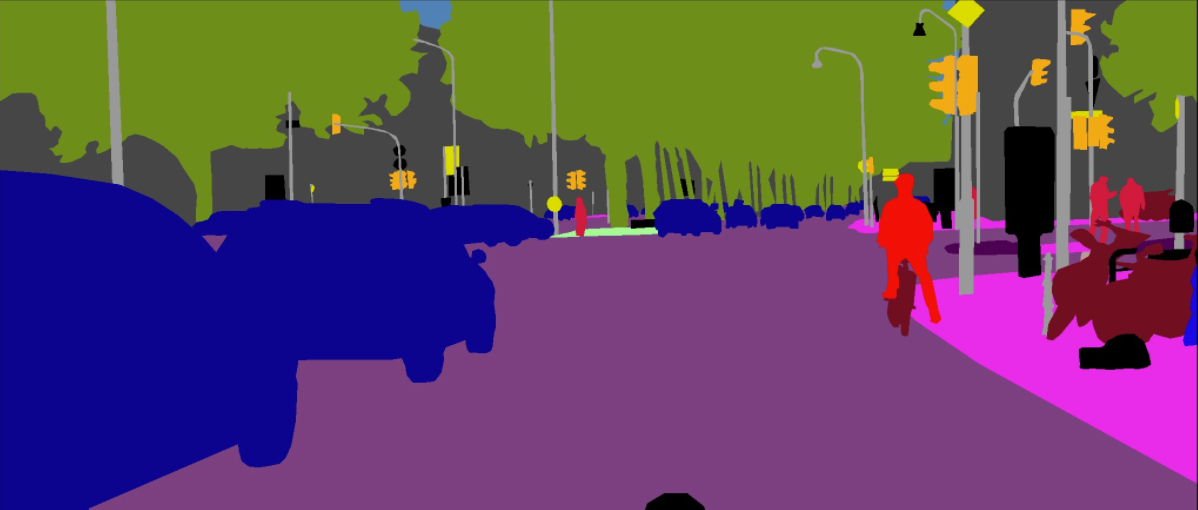### 2.2.提出解决方案¶

#### 2.2.1.用级联的方式设计了空洞卷积模块¶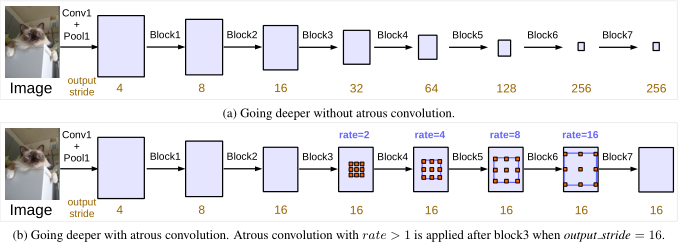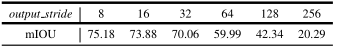#### Atrous Spatial Pyramid Pooling(ASPP):¶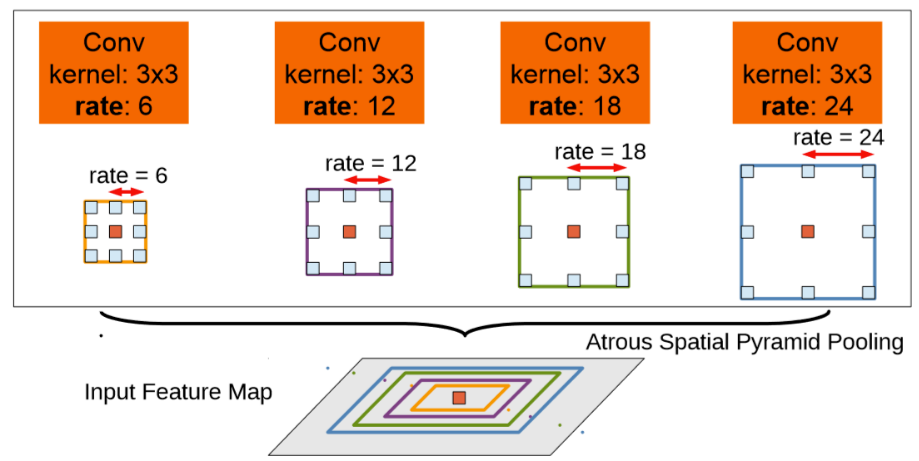ASPPModule代码：

class ASPPModule(nn.Layer):
"""
Atrous Spatial Pyramid Pooling.
Args:
aspp_ratios (tuple): The dilation rate using in ASSP module.
in_channels (int): The number of input channels.
out_channels (int): The number of output channels.
align_corners (bool): An argument of F.interpolate. It should be set to False when the output size of feature
is even, e.g. 1024x512, otherwise it is True, e.g. 769x769.
use_sep_conv (bool, optional): If using separable conv in ASPP module. Default: False.
image_pooling (bool, optional): If augmented with image-level features. Default: False
"""

def __init__(self,
aspp_ratios,
in_channels,
out_channels,
align_corners,
use_sep_conv=False,
image_pooling=False,
data_format='NCHW'):
super().__init__()

self.align_corners = align_corners
self.data_format = data_format
self.aspp_blocks = nn.LayerList()

for ratio in aspp_ratios:
if use_sep_conv and ratio > 1:
conv_func = layers.SeparableConvBNReLU
else:
conv_func = layers.ConvBNReLU

block = conv_func(
in_channels=in_channels,
out_channels=out_channels,
kernel_size=1 if ratio == 1 else 3,
dilation=ratio,
padding=0 if ratio == 1 else ratio,
data_format=data_format)
self.aspp_blocks.append(block)

out_size = len(self.aspp_blocks)

if image_pooling:
self.global_avg_pool = nn.Sequential(
output_size=(1, 1), data_format=data_format),
layers.ConvBNReLU(
in_channels,
out_channels,
kernel_size=1,
bias_attr=False,
data_format=data_format))
out_size += 1
self.image_pooling = image_pooling

self.conv_bn_relu = layers.ConvBNReLU(
in_channels=out_channels * out_size,
out_channels=out_channels,
kernel_size=1,
data_format=data_format)

self.dropout = nn.Dropout(p=0.1)  # drop rate

def forward(self, x):
outputs = []
if self.data_format == 'NCHW':
axis = 1
else:
axis = -1
for block in self.aspp_blocks:
y = block(x)
y = F.interpolate(
y,
interpolate_shape,
mode='bilinear',
align_corners=self.align_corners,
data_format=self.data_format)
outputs.append(y)

if self.image_pooling:
img_avg = self.global_avg_pool(x)
img_avg = F.interpolate(
img_avg,
interpolate_shape,
mode='bilinear',
align_corners=self.align_corners,
data_format=self.data_format)
outputs.append(img_avg)

x = self.conv_bn_relu(x)
x = self.dropout(x)

return x


#### 2.2.4.将全局上下文信息纳入模型¶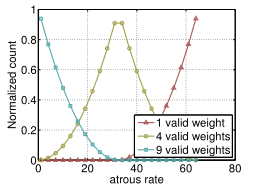• (a)一个 $$1~\times 1$$的卷积与三个$$3~\times 3$$$$rates = (6,12,18)$$的空洞卷积，滤波器数量都为256，包含BN层。针对output_stride=16的情况

• (b)图像级特征，如图5所示。当output_stride=8时，加倍了采样率。然后将所有分支的特征图通过一个$$1~\times 1$$卷积(有256个滤波器和BN)concatenate起来，送入最后的1×1卷积以产生最终分数.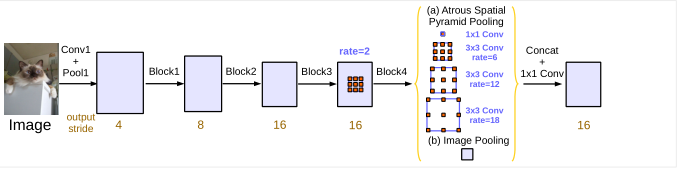2.2.5.取消DenseCRF

CRF

CRF的全称是Conditional Random Field.它的形式如下所示：

${\rm{P}}(Y|X) = \frac{1}{{Z(X)}}\tilde P(Y,X)$
$\tilde P(Y,X) = \exp (\sum\limits_i {{w_i} * {f_i}(Y,X)} )$
$Z(X) = \sum\limits_Y {\exp (\sum\limits_i {{w_i} * {f_i}(Y,X)} )}$

$\tilde P(Y,X) = \exp (\sum\limits_i {{f_1}({X_i},{Y_i}) + {f_2}({Y_i},{Y_{i + 1}})} )$

Dense CRF

$E(x) = \sum\limits_i {{\varphi _u}({x_i}) + } \sum\limits_{i < j} {{\varphi _p}({x_i},{x_j})}$

${\varphi _p}({x_i},{x_j}) = \mu ({x_i},{x_j})\sum\limits_{m = 1}^K {{w^{(m)}}{k^{(m)}}({f_i},{f_j})}$

$k(m)({f_i},{f_j}) = {w^{(1)}}\exp ( - \frac{{{{\left| {{p_i} - {p_j}} \right|}^2}}}{{2\theta _\alpha ^2}} - \frac{{{{\left| {{I_i} - {I_j}} \right|}^2}}}{{2\theta _\beta ^2}}) + {w^{(2)}}\exp ( - \frac{{{{\left| {{p_i} - {p_j}} \right|}^2}}}{{2\theta _\gamma ^2}})$

appearance kernel里面的p表示像素的位置——position，我们的图像是2维的，那么position就有2维。I表示图像的像素值——Intensity，我们的图像是彩色的，那么Intensity就有3维。分式下面的两个参数无论从位置还是长相都像高斯分布中的方差，这里的含义也差不多的。

DeepLabV3 取消DenseCRF的原因

### 2.3.训练策略¶

#### 2.3.1.Learning rate policy:¶

• 采用poly策略， 在初始学习率基础上乘$${(1 - \frac{{iter}}{{\max iter}})^{power}}$$,其中$$power = 0.9$$

#### 2.3.2.Crop size:¶

• 在Pascal VOC 2012数据集的训练和测试中我们采用了513的裁剪尺寸。

#### 2.3.3.Batch normalization：¶

• 我们在ResNet之上添加的模块都包括BN层

• 当output_stride=16时，采用batchsize=16，同时BN层的参数做参数衰减0.9997。

• 在增强的数据集上，以初始学习率0.007训练30K后，冻结BN层参数，然后采用output_stride=8，再使用初始学习率0.001在PASCAL官方的数据集上训练30K。

• 训练output_stride=16比output_stride=8要快很多，因为其中间的特征映射在空间上小四倍。但output_stride=16在特征映射上相对粗糙，快是因为牺牲了精度。

#### 2.3.4.Upsampling logits:¶

• 在先前的工作上，我们是将output_stride=8的输出与Ground Truth下采样8倍做比较。

• 现在我们发现保持Ground Truth更重要，故我们是将最终的输出上采样8倍与完整的Ground Truth比较。

### 2.4.主要代码¶

DeepLabV3

class DeepLabV3(nn.Layer):
"""
The original article refers to
Liang-Chieh Chen, et, al. "Rethinking Atrous Convolution for Semantic Image Segmentation"
(https://arxiv.org/pdf/1706.05587.pdf).
Args:
"""

def __init__(self,
num_classes, #分割类别数
backbone, # 主干网络
backbone_indices=(3, ),
aspp_ratios=(1, 6, 12, 18), # aspp 空洞卷积率
aspp_out_channels=256, # aspp输出通道数
align_corners=False, # 是否对齐
pretrained=None): # 是否预处理
super().__init__()

self.backbone = backbone #主干网络
backbone_channels = [
backbone.feat_channels[i] for i in backbone_indices
]
#定义头模块
backbone_channels, aspp_ratios,
aspp_out_channels, align_corners)
self.align_corners = align_corners
self.pretrained = pretrained
self.init_weight() # 初始化权重

def forward(self, x):
feat_list = self.backbone(x)
return [
# 执行上采样，填充方式使用‘bilinear’
F.interpolate(
logit,
mode='bilinear',
align_corners=self.align_corners) for logit in logit_list
]

def init_weight(self):
if self.pretrained is not None:



class DeepLabV3Head(nn.Layer):
"""
Args:
"""

def __init__(self, num_classes, backbone_indices, backbone_channels,
aspp_ratios, aspp_out_channels, align_corners):
super().__init__()
#定义ASPP模块
self.aspp = layers.ASPPModule(
aspp_ratios,
backbone_channels,
aspp_out_channels,
align_corners,
use_sep_conv=False,
image_pooling=True)
#定义分类头
self.cls = nn.Conv2D(
in_channels=aspp_out_channels,
out_channels=num_classes,
kernel_size=1)

self.backbone_indices = backbone_indices

def forward(self, feat_list):
logit_list = []
x = feat_list[self.backbone_indices]
x = self.aspp(x)
logit = self.cls(x)
logit_list.append(logit)

return logit_list



## 3.实验结果¶

ResNet50：

• 当output_stride为256时，由于严重的信号抽取，性能相比其他output_stride大大的下降了。

• 当使用不同采样率的空洞卷积时，性能上升，这说明了语义分割中使用空洞卷积的必要性。

ResNet-50 vs. ResNet-101: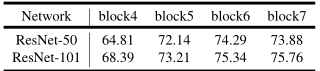• 当block增加，性能也随之增加。

• 随着添加更多的block，提升变得更小。

• 值得注意的是，ResNet-50使用block7会稍微降低性能，同时ResNet-101使用后仍然可以提升性能。

Multi-grid: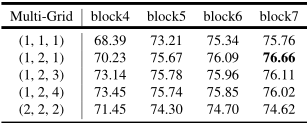• 应用Multi-gird策略通常比单倍数 $$({r_1},{r_2},{r_3}) = (1,1,1)$$效果要好

• 简单的提升倍数例如$$({r_1},{r_2},{r_3}) = (2,2,2)$$是无效的

• 增加网络深度再配合Multi-gird可以提升性能。图中最好的模型即block7下 $$({r_1},{r_2},{r_3}) = (1,2,1)$$

Inference strategy on val set: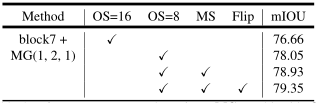• 评估时output_stride=8比output_stride=16性能提高了1.39%。

• 使用多尺度输入(scales={0.5, 0.75, 1.0, 1.25, 1.5, 1.75})和左-右翻转，进行数据增强后，进一步提高了性能。

• 最后，计算每个尺度和翻转图像的平均概率来作为最终结果。

Atrous Spatial Pyramid Pooling:

DeepLab V3的ASPP模块与DeepLab V2的主要区别在于，增加了BN层，增加了图像级别的特征。表5记录了ASPP模块block4使用multi-grid策略和图像级特征后的效果。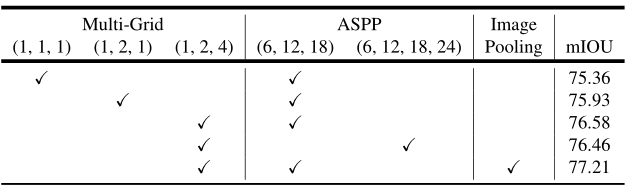Inference strategy on val set: